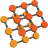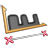You are here: Start » AVL.NET » Surface Features

# Surface Features

Icon Name DescriptionSurfaceArea Computes the surface area of given surface.SurfaceBoundingBox Computes the bounding box 3D of given surface.SurfaceBoundingBox_OrNil Computes the bounding box 3D of given surface; returns NIL if no valid point is present.SurfaceFlatness Computes the surface flatness i.e. how thick the surface is according to the input plane.SurfaceLocalMaxima Detection of characteristic points, usually after some surface transformations.SurfaceLocalMinima Detection of characteristic points, usually after some surface transformations.SurfaceMassCenter Computes the mass center of surface points.SurfaceMassCenter_OrNil Computes the mass center of surface points; returns NIL if the surface is empty in the given region.SurfaceMaximalPoint Finds the surface point with maximal Z coordinate.SurfaceMedian Finds the median Z coordinate of the surface points.SurfaceMedian_OrNil Finds the median Z coordinate of the surface points; returns NIL if the surface is empty in the given region.SurfaceMinimalPoint Finds the surface point with minimal Z coordinate.SurfaceProfileAlongPath This is the first step of all 1D Edge Detection 3D operations. Here available for direct use by the user.SurfaceToPlaneDistanceImage Computes the image of the distances of the input surface points from a given plane.SurfaceValidPointsRegion Computes region of locations where points are valid in a surface and where they are invalid.SurfaceVolume Computes the volume of given surface.